# All Algebra Worksheets

Here you can find all our GCSE algebra worksheets for secondary schools.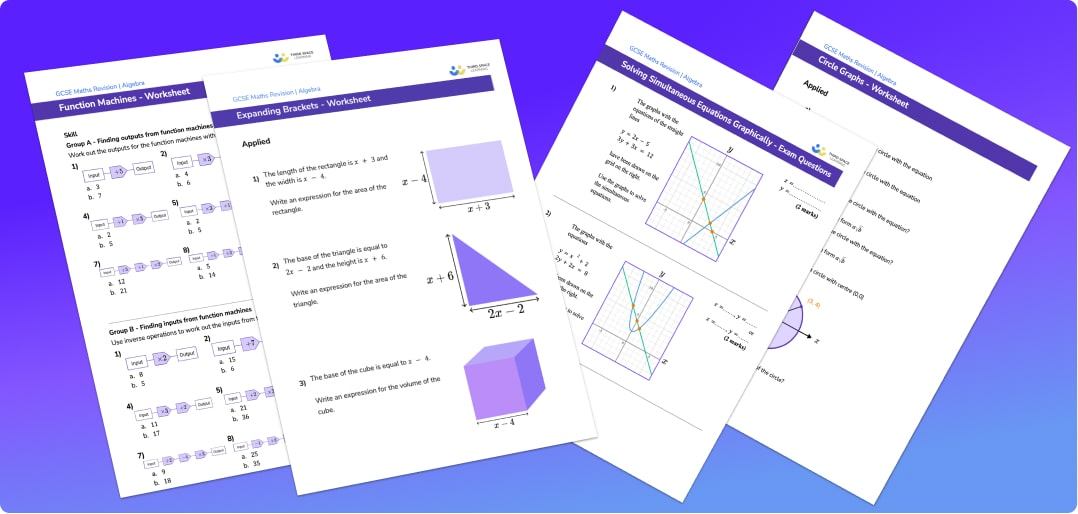What can you expect from the algebra worksheets?

The free algebra worksheets comprise functional and applied reasoning questions, practice questions, exam style questions and word problems.

These printable maths worksheets are suitable for use as part of GCSE maths revision for all major exam boards including Edexcel, AQA and OCR.

Designed to be used within our step-by-step GCSE maths revision lessons.

Mark scheme and answers included for every free algebra worksheet.

Filter by:

Algebra

Geometry

Number

Probability

Ratio and Proportion

Statistics

Mixed

Algebraic expressions

Simplifying expressions

Expanding brackets

Factorising

Equations

Solving equations

Rearranging equations

Simultaneous equations

Maths formulas

Interpreting graphs

Types of graphs

Inequalities

Sequences

Functions in algebra

Laws of indices

Mathematical proof

2D Shapes

Polygons

Symmetry

Area

How to work out perimeter

Circles, sectors and arcs

Angles

Angle rules

Angles in polygons

Angles in parallel lines

3D shapes

How to calculate volume

Prism

Cuboid

Triangular prism

Sphere

Hemisphere shape

Cone

Cylinder

Pyramid

Pythagoras theorem

Trigonometry

Circle theorems

Vectors

Loci And Construction

Transformations

Congruence and similarity

Types of Graphs

Interpreting Graphs

Arithmetic

Rounding numbers

Factors, multiples and primes

Negative numbers

Fractions, decimals and percentages

Fractions

Decimals

Percentages

Comparing fractions decimals and percentages

Powers and roots

Standard form

Simple interest and compound interest

Types of numbers

Surds

Describing probability

Probability distribution

How to calculate probability

Combined events probability

Ratio

Proportion

Compound measures

Scale maths

Units of measurement

Conversion graphs

Rate of change

Types of data

Mean, median, mode

Representing data

Frequency table

Cumulative frequency

Histogram

Types of sampling methods

Clear filter

## How to use the algebra worksheets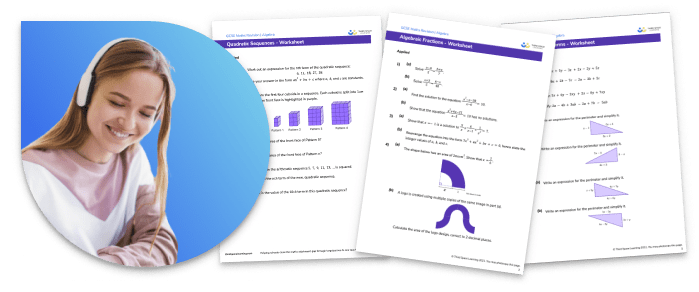Students can practise solving equations, including one-step and two-step algebraic equations by using the inverse of mathematical operations such as addition and subtraction and applying the order of operations.

By working with whole numbers (integers), fractions, decimals and percentages they can answer a variety of algebraic multi-step problems.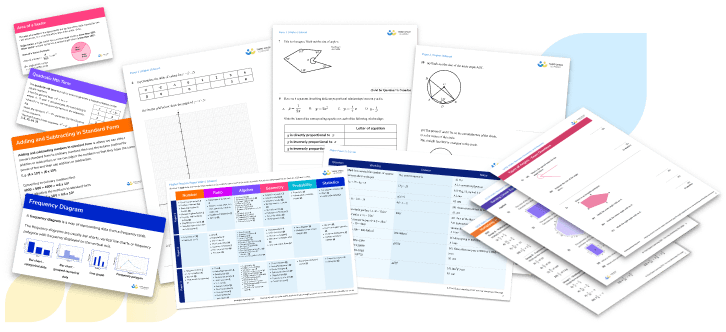In order to solve equations involving polynomials such as quadratic equations, students may need to use the quadratic formula and use square roots and exponents.

Students will also practise using graphs along with defining unknowns and missing numbers.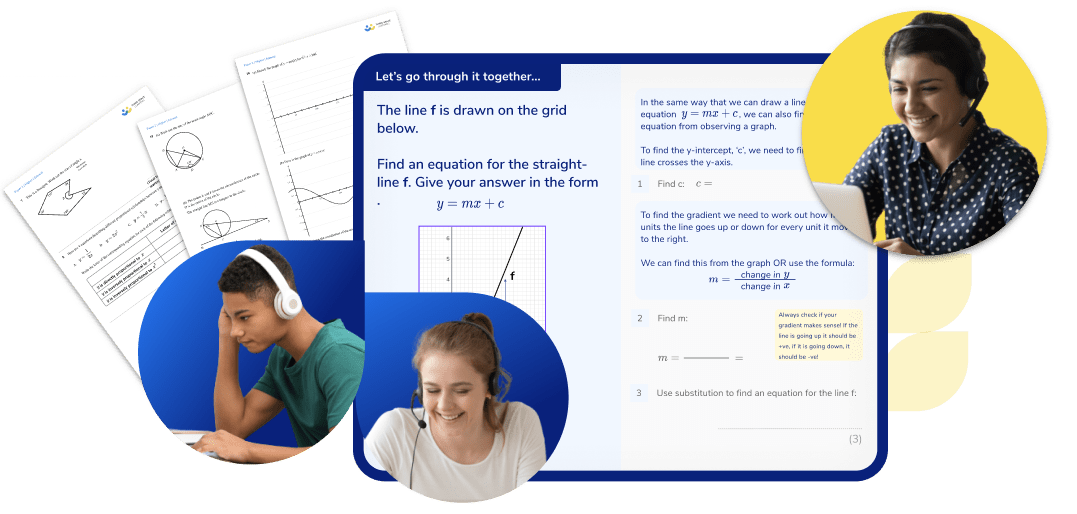You can find further GCSE maths resources within these step by step Algebra lessons.

For any students struggling with Algebra, our GCSE maths revision programme of online tuition provides the one to one personalised support they need to develop understanding and make rapid progress.

## Can't find what you're looking for?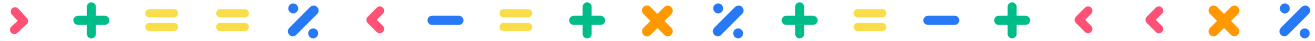## Teaching support from the UK’s largest provider of in-school maths tuition

At Third Space Learning, we’re on a mission to help teachers like you shape your students into confident, able mathematicians.

Request a quote